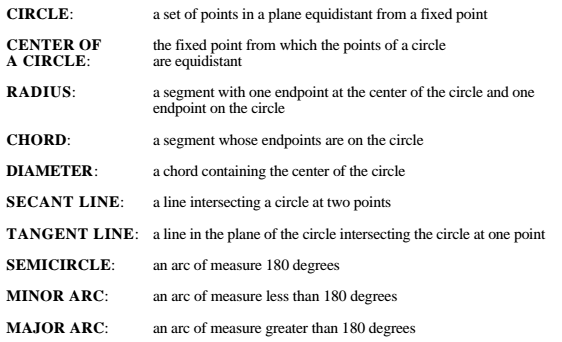# Angle Arc Basics

Author:
Aja Wright
Topic:
Angles

## Angle Arc Basics Introduction

What is the purpose of this set of seven investigations?
• You will learn the relationships between angles and arcs.
• You will develop conjectures and formulas about angles and arcs.
• You will learn and/or review vocabulary related to circular geometry.
• You will practice using GeoGebra.

## Important Vocabulary for you to know:## Central and Inscribed Angles

How are the center of the circle and the vertex of the angle related?

1. In your own words, describe the relationship between the measure of the central angle and the arc.

Express the relationship of the central angle, A, to the intercepted arc, arcDC as a formula. Be sure to define your variables.

In your own words, describe the relationship between the arc and the angle measure for the INSCRIBED angle, E.

Express the relationship of the inscribed angle, E, to the intercepted arc, arcDC as a formula. Be sure to define your variables.

## Inside Angles--when lines cross inside the circle to form angles

In your own words, describe the relationship between the measure of the inside angle and the measures of its two intercepted arcs. (As a hint, this relationship involves two arithmetic operations.)

Use variables to write the relationship of the measure of the inside angle to the measures of the two arcs.

In your own words, describe the relationship between the measure of an outside angle and the measures of its intercepted arcs.

Write the relationship between the intercepted arcs and the outside angle as an equation. Call the larger arc aL and the smaller one aS, and you can call the angle E.

How is the measure of this type of angle related to its intercepted arc?

To which arc is angle CED related?

To which arc is angle CFD related?

In your own words, explain why the relationship between the measures of angles CED and CFD is true.

In Part I you investigated four types of angles: central, inscribed, inside, and outside. Which of these types of angles is angle CDB?

There are three types of arcs: minor arc, semicircle, major arc. Which of these types of arcs is arc CDB?

Complete this sentence: An angle inscribed in a ________________ is _____________________.

1. (Hint: Put a type of arc in the first blank.)

Why is an angle inscribed in a semi-circle always 90?

Find the sum of <BCD + <BED.

Find the sum of <CBE + <CDE.

What is the name for the relationship between <BCD and <BED? Or for the relationship between <CBE and <CDE?

Complete this sentence: If a ______________ (name of polygon) is inscribed in a circle, the ___________ (type of angles) are ____________________(some angle relationship).

In your own words, explain why it is true that when you have an inscribed quadrilateral, then the opposite angles are supplementary.